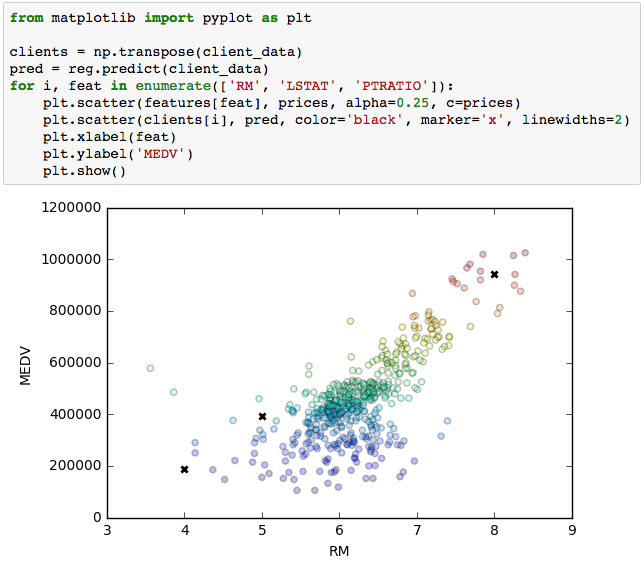A very simple introduction to Matplotlib in Python. It tries to present fundamental concepts without clinging to many details. If you have time I highly recommend you another post which is much more conceptual, detailed and illustrated than mine: “Artist” in matplotlib.

import matplotlib.pyplot as plt

Basics: Figures and Axes

The most important thing that you have to know is that Matplotlib has two main classes for plotting curves and setting up configurations: Figure and Axes. In this section we present these objects and its main methods.

Figure

The Figure object is a major object that contains all of the plot elements (including the Axes object, which we will see next). It can be accessed in the following manners:

fig = plt.figure() # returns a Figure instance

The last line is a shortcut for getting the current figure and its axes; see these answers for more details.

Configurations such as saving and displaying the figure are set at the figure-level:

fig.savefig('filepath.png') # saves figure
fig.show() # displays figure

Axes

Axes are the objects that have plotting methods. Each figure can contain multiple axes, thus allowing you to make multiple plots on the same figure. They are created and accessed from the figure object:

# create new axes
ax1 = fig.subplots() # add a set of subplots to this figure (default 1)

# get existing axes
axs = fig.axes # list of axes in `fig`

Once you have the axis object that you want to plot a curve into, the only thing you need to do is to call the plotting method:

ax1.plot(x, y)

There are many different kinds of plotting methods (which we will not cover), here are a few:

• ax.plot Lines and/or Markers
• ax.bar Bars chart
• ax.hist Histogram
• plt.scatter Scatter (has capability to render a different size and/or color for each point, in contrast to plot)

Shortcuts: the Pyplot interface

To make things easier, Matplotlib has shortcuts for the methods mentioned above directly through pyplot. That is, lots of methods can be called from pyplot, regardless of whether they would normally be called from a Figure or an Axis object:

import matplotlib.pyplot as plt

# Example 1
plt.plot([1,2,3])
plt.savefig('filepath.png')
plt.show()

# Example 2
fig, ax1 = plt.subplots() # get figure and 1 axes (already added to fig)
...

Axis labels, Legends, and Title

Legend, title and axis labels should be set in the axis object:

# TITLE AND AXIS LABELS
ax.set(title='title', xlabel='xlabel', ylabel='ylabel')
# or
ax.set_title('title')
ax.set_xlabel('xlabel')
ax.set_ylabel('ylabel')

# LEGEND
# add labels to curves
ax.plot(xa, ya, label='Algorithm A')
ax.plot(xb, yb, label='Algorithm B')

# call legend function
ax.legend()

Of course, Matplotlib has shortcuts for these methods directly through pyplot as well, in the case you are dealing with a single plot:

# shortcuts
plt.title('title')
plt.xlabel('xlabel')
plt.ylabel('ylabel')
plt.legend()

Subplots

In Matplotlib, the subplot functionality allows you to specify where the axes should be situated (in the figure) according to a subplot grid.

ax1 = fig.add_subplot(221) # creates an axis in the position specified (row-col-num)
ax2 = fig.add_subplot(222) # creates an axis in the position specified (row-col-num)
ax3 = fig.add_subplot(223) # creates an axis in the position specified (row-col-num)
ax4 = fig.add_subplot(224) # creates an axis in the position specified (row-col-num)
# or
[[ax1, ax2], [ax3, ax4]] = fig.subplots(nrows=2, ncols=2) # add a set of subplots to this figure
# or
fig, [[ax1, ax2], [ax3, ax4]] = plt.subplots(nrows=2, ncols=2) # shortcut for getting both figure and (all) axes

After getting the axes, you can call the plotting method of your choice for each one.

Notice that these functions do not impose a grid to the figure, however. What they do is to simply create new axes that occupy specific positions on the figure. Because of that, you can create subplots with varying widths and heights in the same figure. Some examples are covered in this tutorial.

If you don’t want to be restricted to a grid, check out the add_axes method. Its differences to the add_subplot method are well discussed here.

Decoration

Usually configuration of the curves, bars, histograms, etc. are done in the respective plotting function:

plt.plot(x, y, color='green', marker='o', linestyle='dashed',
linewidth=2, markersize=12)

Check out the docs for more details:

Specific tips

• The parameter alpha is usually the transparency of the curve.
• Use plt.tight_layout() when your subplots have titles

Examples

Highlighting specific data points in a scatter plotColor warmth in scatter plotTrue vs predicted data points